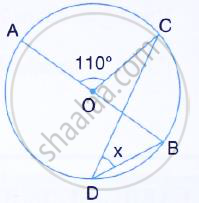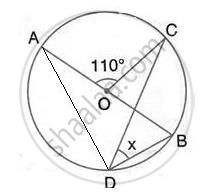Share

In the Figure, Given Below, Aob is a Diameter of the Circle and ∠Aoc = 110°. Find ∠Bdc. - ICSE Class 10 - Mathematics

ConceptChord Properties - a Straight Line Drawn from the Center of a Circle to Bisect a Chord Which is Not a Diameter is at Right Angles to the Chord

Question

In the figure, given below, AOB is a diameter of the circle and ∠AOC = 110°. Find ∠BDC.SolutionHere, ∠ADC = 1/2∠AOC = 1/2xx 110° = 55°